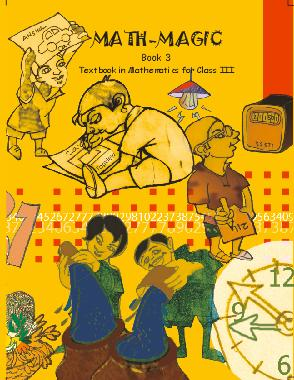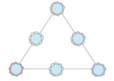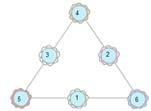# NCERT Solutions for Class 3 Maths Give and take## myCBSEguide App

CBSE, NCERT, JEE Main, NEET-UG, NDA, Exam Papers, Question Bank, NCERT Solutions, Exemplars, Revision Notes, Free Videos, MCQ Tests & more.

NCERT solutions for Class 3 Mathematics## NCERT Solutions for Class 3 Maths Give and take

CHAPTER  3 Class 3 Maths Give and take

Ans. 10 steps.

Ans. 3 steps.

### 3. Try these on Kittu’s home:

(a) 10 less than 34 is 24.

Ans. 24.

(b) 53 – 20 = ___

Ans. 53 – 20 = 33.

(c) 11 more than 31 is ___.

Ans. 42.

(d) 11 less than 66 is __.

Ans. 55.

(e) 62 + 13 = ___.

Ans. 75.

(f) 23 less than 89 is __.

Ans. 23 less than 89 is 66.

(g) 10 and 40 more than is ___.

Ans. 10 and 40 more than is 50.

(h) 9 added to 28 gives ___.

Ans. 37.

(i) The sum of 9 and 44 is ___.

Ans. The sum of 9 and 44 is 53.

(j) Reducing 98 by 34 gives ___.

Ans. 64.

(k) 4 and 37 more is __.

Ans. 4 and 37 more is 41.

(l) Take 35 away from 83. We get __.

Ans. We get 48.

(a) 37 + 9 = _______

Ans. 46.

(b) 65 – 30 = _____

Ans. 35.

(c) _____= 46 + 21

Ans. 67.

(d) ___ = 87 – 30

Ans. 57.

(e) 66 – ___ = 11

Ans. 55.

(f) 36 = __ + 9

Ans. 27.

(g) 45 + ___ = 99

Ans. 54.

(h) 40 + ___ = 76

Ans. 36.

(i) __ + 26 = 75

Ans. 49.

(j) 98 = __ + 50

Ans. 48.

(k) ___ – 21 = 35

Ans. 14.

(l) 57 – ___ = 20

Ans. 37.

### 5. Can you do it another way? Show how.

(a) 37 + 21 = 30 + 7 + 20 + 1

Ans. 37 + 21 = 30 + 7 + 20 + 1

= 50 + 7 + 1

= 50 + 8 = 58

(b) 73 + 24 = 70 + 3 + 20 + 4

Ans. 73 + 24 = 70 + 3 + 20 + 4

= 90 + 3 + 4

= 90 + 7 = 97

(c) 56 + 21 = 50 + 6 + 20 + 1

Ans. 56 + 21 = 50 + 6 + 20 +1

= 70 + 6 + 1

= 70 + 7 = 77

### 6. Now work out the steps in your mind. Write the answers directly in the boxes.

(a)  33 +42 = ___

Ans. 75.

(b) __ = 33+ 27

Ans. 60.

(c) 55 + 25 = ___

Ans. 80.

(d) 19 + 61 = ___

Ans. 80.

(e) ___ = 34 + 63.

Ans. 97.

(f) 67 + 25 = ___

Ans.  92.

(g) __ = 48 + 42

Ans. 90

(h)  ___ = 53 +64

Ans. 117.

(i) 72 +56 = ___

Ans. 128.

### 7. A Shopkeeper Rafi had 153 candles. Paras gave him 237 more candles. How many candles does Rafi have now?

Ans.

 100 10 1 2 3 7 + 1 5 3 Sum 3 9 0

### 8. Work out the following story problems in the same way. Read each problem and say it in your word. Guess the answer before writing it.

(a) A train compartment is carrying 132 people.  Another compartment is carrying 129 people. In all, how many people are there in both the compartments.

Ans.

 100 10 1 People in compartment 1 3 2 People in other compartment + 1 2 9 Sum 2 6 1

(b) Shannu found 138 pebbles. Karim found 44 pebbles. How many pebbles did they find in all?

Ans.

 100 10 1 1 3 8 + 4 4 1 8 2

(c) A teacher kept a note of which fruits students like in her school. This is what she found:

 Students Oranges Mangoes Total Girls 136 240 Boys 128 243 Total

(i) How many students in the school like oranges?

Ans.

 Students Oranges Mangoes Total Girls 136 240 376 Boys 128 243 371 Total 264 483 747

Number of students who like oranges = 136 + 128 = 264.

(ii) How many students in the school like mangoes?

Ans. Number of students in the school like mangoes = 240 + 243 = 483.

(iii) Altogether, how many students are there in the school?

Ans. Total number of students = 376 + 371 = 747.

(iv) Is the number of girls more than 350 or less than 350?

Ans. Number of girls is more than 350. It is 376.

### 9. Practice Time:

(a)

 3 4 5 + 5 2

Ans.

 3 4 5 + 5 2 3 9 7

(b)

 4 9 2 + 2 9

Ans.

 4 9 2 + 2 9 5 2 1

(c)

 2 4 5 + 9 3

Ans.

 2 4 5 + 9 3 3 3 8

(d)

 6 4 3 + 3 4 5

Ans.

 6 4 3 + 3 4 5 9 8 8

(e)

 7 5 0 + 2 1 9

Ans.

 7 5 0 + 2 1 9 9 6 9

(f)

 3 1 9 + 8 2 3

Ans.

 3 1 9 + 8 2 3 1 1 4 2

(g)

 8 0 4 + 4 0 6

Ans.

 8 0 4 + 4 0 6 1 2 1 0

(h)

 3 6 3 + 4 5 6

Ans.

 3 6 3 + 4 5 6 8 1 9

(i)

 3 6 3 + 4 5 6

Ans.

 3 6 3 + 4 5 6 6 7 5

(j)

 6 8 4 + 2 3 2

Ans.

 6 8 4 + 2 3 2 9 1 6

Class 3 Maths Give and take

### 10. Work out for different ways to write the numbers.

(a)

 78 + + 30 + +

Ans.

 78 70 + 8 50+28 30+48 78+0

(b)

 83 + +43 + +

Ans.

 83 80+3 40+43 50+43 83+0

(c)

 99 + +39 + +

Ans.

 99 90+9 60+39 50+49 99+0

(d)

 102 + + + +50

Ans.

 102 100+2 90+12 80+22 52+50

(e)

 168 + + +68 +

Ans.

 168 160+8 18+150 100+68 120+48

### 11. Can you solve this puzzle? Write the numbers 1,2, 3, 4,5, 6 in the circles, so that the sum of the numbers on each side of the figure is 12.Ans.Upper train have

= (19 – 5) + (6 – 15) + (7 – 3) + (6 – 10) + (4 – 20) + (1 – 7) + (5 – 4)

= 14 – 9 + 4 – 4 – 16 – 6 + 1

= 19 – 35 = -16

Lower train have

= (17 – 20) + (3 – 19) + (9 + 12) + (9 – 20)

= -3 – 16 + 21 – 11

= -30 + 21 = -30 + 21 = -9

Class 3 Maths Give and take

### 12. Card game:

(a)

 50 70 20

 50 20 70

Ans.

 50 70 20 50 + 20 = 70 20 + 50 = 70

 50 20 70 70 – 50 = 20 70 – 20 = 50

(b)

 30 42 12

 30 42 12

Ans.

 30 42 12 30 + 12 = 42 12 + 30 = 42

 30 42 12 42 – 12 = 30 42 – 30 = 12

## NCERT solutions for Class 3 Mathematics Chapter 3 Give and take

NCERT Solutions Class 3 Maths Give and take PDF (Download) Free from myCBSEguide app and myCBSEguide website. Ncert solution class 3 Maths includes text book solutions from Class 3 Maths Book . NCERT Solutions for CBSE Class  Maths have total 14 chapters. 3 Maths NCERT Solutions in PDF for free Download on our website. Ncert Maths class 3 solutions PDF and Maths ncert class 3 PDF solutions with latest modifications and as per the latest CBSE syllabus are only available in myCBSEguide.

## CBSE app for Students

To download NCERT Solutions for Class 3 EVS Hindi English, Maths do check myCBSEguide app or website. myCBSEguide provides sample papers with solution, test papers for chapter-wise practice, NCERT solutions, NCERT Exemplar solutions, quick revision notes for ready reference, CBSE guess papers and CBSE important question papers. Sample Paper all are made available through the best app for CBSE students and myCBSEguide website.